### IMO Shortlist 2017 problem G5

Kvaliteta:
Avg: 0,0
Težina:
Avg: 8,0

Let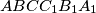$ABCC_1B_1A_1$ be a convex hexagon such that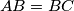$AB=BC$, and suppose that the line segments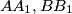$AA_1, BB_1$, and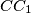$CC_1$ have the same perpendicular bisector. Let the diagonals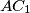$AC_1$ and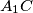$A_1C$ meet at$D$, and denote by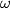$\omega$ the circle$ABC$. Let$\omega$ intersect the circle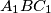$A_1BC_1$ again at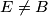$E \neq B$. Prove that the lines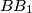$BB_1$ and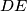$DE$ intersect on$\omega$.

Izvor: https://www.imo-official.org/problems/IMO2017SL.pdf